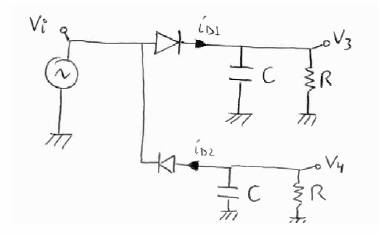# Circuit with Diodes, Capacitors, and Resistors

• Engineering

## Homework Statement

Compute V3(t)-V4(t). The capacitors are initially discharged, the voltage source is turned on at t = 0, and the diodes’ forward voltage drop is negligible (Vf = 0). What is the value of V3(10s) – V4(10s)?

Vi=4sin(2∏t)
RC= 0.1s## Homework Equations

i (over capacitor) = CdV/dt
i (over resistor) = V/R
Assume Diode is either on (id>0) or off (id=0)

## The Attempt at a Solution

I used the node method: node above the top capacitor has voltage V1 and node above the bottom capacitor has voltage V2. Using KCL at those two nodes, I found id1=(CdV1/dt)+(V3/R) and id2=-(CdV2/dt)-(V4/R). Unknowns are id1, id2, V1, V2, V3, and V4.

Based on whether I assume a diode is on/off I can assume V1=Vi or V2=Vi and that id1=0 or id2=0, so that eliminates two unknowns. I'm still left with 4 variables though and can't see any other equations to solve for them. I was thinking use the node between the two diodes and the voltage source, but I don't know the current coming off the source. And there aren't any closed loops for me to use KVL.

Simon Bridge
Homework Helper
Look at what the half-wave effect has - capacitors are charging for half a cycle and then discharging the other half. Compare the polarity of upper and lower caps when semi-charged.

Have you used the expression for v(t) input that results from the diodes?

I'm not too sure what you mean by comparing the polarity.

From the diodes, I only know that if D1 is on (and D2 is off), V1=Vi and V2<Vi. If D2 is on (and D1 is off), V2=Vi and Vi<V1. If both are off, V2<Vi<V1. I can sub in Vi but I don't know any other V(t) input I can use in regards to the other voltages.

berkeman
Mentor

## Homework Statement

Compute V3(t)-V4(t). The capacitors are initially discharged, the voltage source is turned on at t = 0, and the diodes’ forward voltage drop is negligible (Vf = 0). What is the value of V3(10s) – V4(10s)?

Vi=4sin(2∏t)
RC= 0.1s## Homework Equations

i (over capacitor) = CdV/dt
i (over resistor) = V/R
Assume Diode is either on (id>0) or off (id=0)

## The Attempt at a Solution

I used the node method: node above the top capacitor has voltage V1 and node above the bottom capacitor has voltage V2. Using KCL at those two nodes, I found id1=(CdV1/dt)+(V3/R) and id2=-(CdV2/dt)-(V4/R). Unknowns are id1, id2, V1, V2, V3, and V4.

Based on whether I assume a diode is on/off I can assume V1=Vi or V2=Vi and that id1=0 or id2=0, so that eliminates two unknowns. I'm still left with 4 variables though and can't see any other equations to solve for them. I was thinking use the node between the two diodes and the voltage source, but I don't know the current coming off the source. And there aren't any closed loops for me to use KVL.

I'm not too sure what you mean by comparing the polarity.

From the diodes, I only know that if D1 is on (and D2 is off), V1=Vi and V2<Vi. If D2 is on (and D1 is off), V2=Vi and Vi<V1. If both are off, V2<Vi<V1. I can sub in Vi but I don't know any other V(t) input I can use in regards to the other voltages.

Hint -- start with the resistor values very high (so the discharge time constant is many seconds. What does each capacitor charge to (that is Simon's hint)? Now, change the time constant to 0.1s -- does that change your answer by much?

Simon Bridge
Homework Helper
The supply if giveing you a waveform of something like: vi(t)=Vsin(wt)
The sketch of this is a sign wave.
This is the wave at each diode.

The top diode means that the circuit after it will only get a particular shape v1(t) driving it.
That shape is not the same as vi(t). It is not a simple "on" vs "off" state either.
You are thinking too algebraically: look for understanding first.
What is that shape? Sketch it. Don't worry about being exact. What does the sketch look like?

Do the same for the effective driving voltage for the lower circuit - what is that?

...and thank you berkman :)

berkeman
Mentor
BTW, the frequency of the excitation is pretty low, so your answer may change by enough to be important

"Vi=4sin(2∏t)"

berkeman
Mentor
Piling on!